# latency and throughput of MAD operation?

Dear all:

From Volkov’s paper,

Vasily Volkov, James W. Demmel, Benchmarking GPUs to Tune Dense Linear Algebra. In SC â€™08: Preceedings of the 2008 ACM/IEEE conference on Supercomputing.

Piscataway, NJ, USA, 2008, IEEE Press.

( can be obtained in the thread http://forums.nvidia.com/index.php?showtopic=89084 )

authors show

(1) average time per instruction in cycles (a, b, c are register) for GPUs in Table 1

Table 1: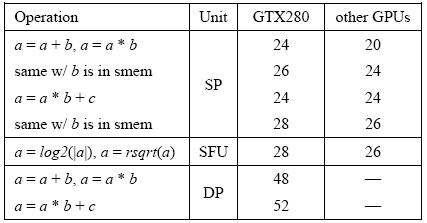(2) average time per instruction in cycles (a, b: register, s[i]: shared memory) in Table 2

Table 2: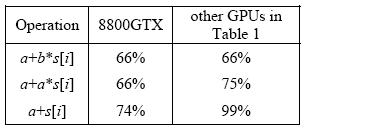I focus on latency and throughput of three instructions on TeslaC1060,

one is “a = a b", one is "a = as[i] + c” and another is “a = a * b + c”.

from decuda program,

“a = a *b” is translated to “MUL dest src1 src2”

“a = a*s[i] + c” is translated to “MAD dest [smem] src2 src3”

“a = a*b + c” is translated to “MAD dest src1 src2 src3” and

From my experiment (show later), I conclude

(1) “a = a*b” has latency = 24.7 cycle and throughput 2.5 ~ 4.1 cycle per warp

(this number is very strange, I will show experimental data later)

(2) “a = a*s[i] + c” has latency 34.6 cycle and throughput 6 cycle per warp.

(this number is good and matches result in Table 2)

(3) “a = a*b + c” has latency 31.5 cycle and throughput = ? (maybe 4 cycle per warp)

latency = 31.5 cycle is larger than 24 cycle in Table 1,

Does anyone have verified these numbers?

The following is my experiment on TeslaC1060.

Step 1: calibrate “a = a*b”

The “CODE 1” sets “a” as register A_reg and “b” as register b_reg and

compute “A_reg = A_reg * b_reg ;” 256 times.

CODE 1: kernel of “a = a *b”

``````static __global__ void MAD_latency(float * data,  timing_stats * timings)

{

unsigned int start_time = 1664;

unsigned int end_time = 1664;

float A_reg = data;

float b_reg = data;

start_time = clock();

#pragma unroll

for( int j = 0; j < 256; j++){

A_reg = A_reg * b_reg ;

}

end_time = clock();

if ( 0 == threadNum ){

}

}
``````

and “DECUDA 1” shows decuda’s result of

``````start_time = clock();

#pragma unroll

for( int j = 0; j < 256; j++){

A_reg = A_reg * b_reg ;

}

end_time = clock();

``````

DECUDA 1: “a = a *b” --> “mul \$r3 \$r3 \$r2”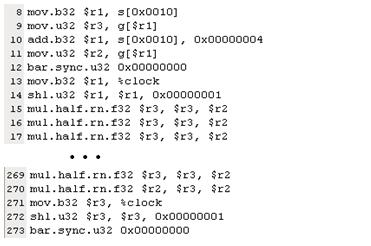when kernel’s execution, we compute average time over 256 per thread (see “code 2”) and

reports minimum time and maximum time in Table 3.

CODE 2: compute average number of cycles per “a = a*b”

``````dim3  grid(1, 1, 1);

// show all time report

int min_time = timings_cpu.end_time - timings_cpu.start_time;

int max_time = min_time;

for( int i = 0; i < NUM_THREADS; i++ ){

int time_gpu = timings_cpu[i].end_time - timings_cpu[i].start_time;

if ( min_time > time_gpu ) { min_time = time_gpu; }

if ( max_time < time_gpu ) { max_time = time_gpu; }

}
``````

Table 3: result of “a = a*b”

From Table 3, NUM_THREADS=1 reports latency = 24.7 cycle, this is consistent with Volkov’s result.

To compute throughput, we define throughput = (average time of the instruction)/(number of warp)

and show throughput in Table 4.

Table 4: throughput of “a = a*b”

Table 4 shows throughput = 2.5 ~ 4.1 cycle per warp, this is very strange

since we have known 24-cycle pipeline latency can be hidden by 6 warps, see Gatt chart in figure 1

Figure 1: pipeline latency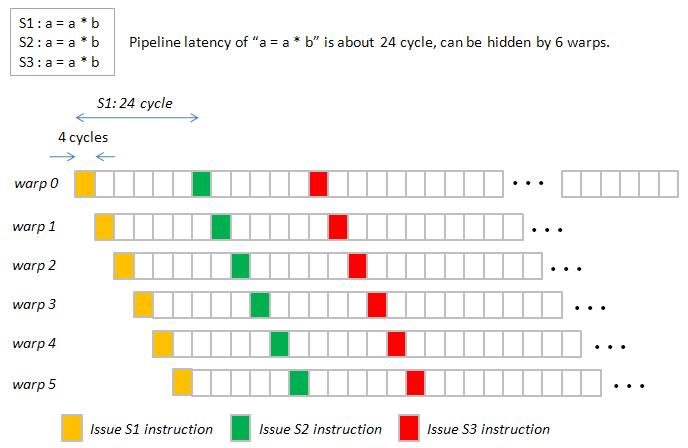and if we invoke more than 6 warps, total time for one instruction is corrected as

total time of one “a=a*b” is (4 cycle) x (number of warps), see figure 2

Figure 2: total time of one “a=a*b”

However when threads = 512, throughput is 2.5 cycle per warp, this is much smaller than 4 cycle per warp.

Remark: From test harness of @SPWorley in the thread

SPWorley uses one block of 192 threads to calibrate “how many clocks it takes”.

Under 192 threads, I will say throughput of “a=a*b” is 4.1 cycle per warp.

Step 2: calibrate “a = a*s[i] + c”

The “CODE 3” sets “a” as register A_reg and “s[i]” as shared memory b[i] and

“c” as register c_reg.

CODE 3: kernel of “a = a*s[i] + c”

``````static __global__ void MAD_latency(float * data,  timing_stats * timings)

{

__shared__ float b[BLOCKSIZE];

unsigned int start_time = 1664;

unsigned int end_time = 1664;

b[j] = data[j];

}

float A_reg = data;

float c_reg = data;

start_time = clock();

#pragma unroll

for( int j = 0; j < 16; j++){

#pragma unroll

for( int i = 0; i < 16; i++){

A_reg = A_reg * b[i] + c_reg;

}

}

end_time = clock();

if ( 0 == threadNum ){

}

}
``````

and “DECUDA 2” shows decuda’s result of

``````start_time = clock();

#pragma unroll

for( int j = 0; j < 16; j++){

#pragma unroll

for( int i = 0; i < 16; i++){

A_reg = A_reg * b[i] + c_reg;

}

}

end_time = clock();

``````

DECUDA 2: “a = a*s[i] + c” --> “mad \$r3 s[…] \$r3 \$r2”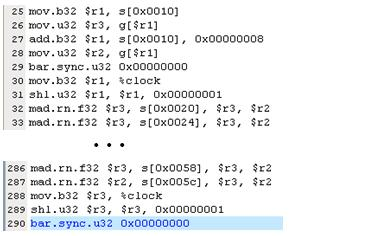experimental result (see Table 5) shows

Latency of “a = a*s[i] + c” = 34.6 cycle

Throughput of “a = a*s[i] + c” is about 6 cycle per warp, this number matches Table 2.

Table 5: result of “a = a*s[i] + c”

Step 3: calibrate “a = a * b + c”

The “CODE 4” sets “a, ,b, c” as register A_reg, b_reg and c_reg.

CODE 4: kernel of “a = a * b + c”

``````static __global__ void MAD_latency(float * data,  timing_stats * timings)

{

unsigned int start_time = 1664;

unsigned int end_time = 1664;

float A_reg = data;

float b_reg = data;

float c_reg = data;

start_time = clock();

#pragma unroll

for( int j = 0; j < BLOCKSIZE * MAXITE; j++){

A_reg = A_reg * b_reg + c_reg;

}

end_time = clock();

if ( 0 == threadNum ){

}

}
``````

and “DECUDA 3” shows decuda’s result of

``````start_time = clock();

#pragma unroll

for( int j = 0; j < BLOCKSIZE * MAXITE; j++){

A_reg = A_reg * b_reg + c_reg;

}

end_time = clock();

``````

DECUDA 3: “a = a * b + c” --> “mad \$r4 \$r4 \$r3 \$r2”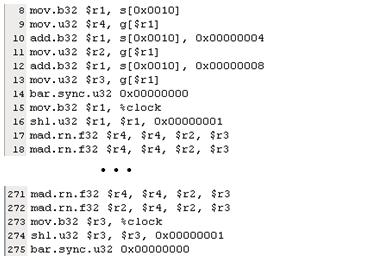Experimental result (see Table 6) shows latency = 31.5 cycle,

however minimum time and maximum time are much different when NUM_THREADS > 256, I don’t know why.

if we focus on NUM_THREADS=192, 224, 256, then throughput is 4 cycle per warp,

and we need 8 warps to hide pipeline latency (=31.5 cycle)

Table 6: result of “a = a * b + c”